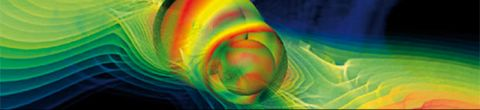The Theoretical MinimumFall, 2013

Building on Professor Susskind’s previous Continuing Studies courses on quantum mechanics, this course will explore the various types of quantum systems that occur in nature, from harmonic oscillators to atoms and molecules, photons, and quantum fields.  Students will learn what it means for an electron to be a fermion and how that leads to the Pauli exclusion principle. They will also learn what it means for a photon to be a boson and how that allows us to build radios and lasers. The strange phenomenon of quantum tunneling will lead to an understanding of how nuclei emit alpha particles and how the same effect predicts that cosmological space can “boil.”  Finally, the course will delve into the world of quantum field theory and the relation between waves and particles.

Lectures in this Course

1. Review of quantum mechanics and introduction to symmetry

The course begins with a brief review of quantum mechanics and the material presented in the core Theoretical Minimum course on the subject. The concepts covered include vector... [more]
2. Symmetry groups and degeneracy

Professor Susskind presents an example of rotational symmetry and derives the angular momentum operator as the generator of this symmetry. He then presents the concept of degenerate states, and shows that any two symmetries that do not commute imply... [more]
3. Atomic orbits and harmonic oscillators

Professor Susskind uses the quantum mechanics of angular momentum derived in the last lecture to develop the Hamiltonian for the central force coulomb potential which describes an atom.  The solution of the Schrödinger equation for this system leads... [more]
4. Spin

Professor Susskind builds on the discussion of quantum harmonic oscillators from the last lecture to derive the higher order energy states and wave functions.  He then moves on to discuss spin states of particles, and introduces the Pauli matrices,... [more]
5. Fermions: a tale of two minus signs

Professor Susskind presents the quantum mechanics of multi-particle systems, and demonstrates that fermions and bosons are distinguished by the two possible solutions to the wave function equation when two particles are swapped.  When two particles... [more]
6. Quantum field theory

Professor Susskind introduces quantum field theory.  Excepting gravity, quantum field theory is our most complete description of the universe.  Each quantum field corresponds to a specific particle type, and is represented by a state vector... [more]
7. Quantum field theory 2

Professor Susskind continues with the presentation of quantum field theory.  He reviews the derivation of the creation and annihilation operators, and then develops the formulas for the energy of a multi-particle system.  This derivation... [more]
8. Second quantization

Professor Susskind answers a question about neutrino mixing and relates the oscillating quantum states of a neutrino to a precessing electron spin in a magnetic field.  He then discusses a recent article about whether an electron is a sphere.  After... [more]
9. Quantum field Hamiltonian

Professor Susskind presents the Hamiltonian for a quantum field, and demonstrates how these Hamiltonians describe particle interactions such as decay and scattering. He then introduces the field theory for fermions by deriving the Dirac equation.... [more]
10. Fermions and the Dirac equation

Professor Susskind closes the course with the presentation of the quantum field theory for spin-1/2 fermions.  This theory is based on the Dirac equation, which, when Dirac developed it in 1928, was the first thory to account fully for special... [more]